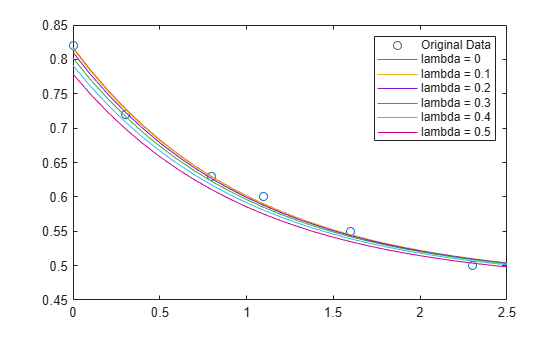# fixed.qrMatrixSolve

Solve system of linear equations Ax = B for x using QR decomposition

Since R2020b

## Syntax

``x = fixed.qrMatrixSolve(A,B)``
``x = fixed.qrMatrixSolve(A,B, outputType)``
``x = fixed.qrMatrixSolve(A,B,outputType,regularizationParameter)``

## Description

example

````x = fixed.qrMatrixSolve(A,B)` solves the system of linear equations Ax = B using QR decomposition.```

example

````x = fixed.qrMatrixSolve(A,B, outputType)` returns the solution to the system of linear equations Ax = B as a variable with the output type specified by `outputType`.```

example

````x = fixed.qrMatrixSolve(A,B,outputType,regularizationParameter)` returns the solution to the system of linear equations $\left[\begin{array}{l}\lambda {I}_{n}\\ A\end{array}\right]x=\left[\begin{array}{l}{0}_{n,p}\\ B\end{array}\right]$where A is an m-by-n matrix, B is an m-by-p matrix, and λ is the regularization parameter.```

## Examples

collapse all

This example shows how to solve a simple system of linear equations $\mathit{Ax}=\mathit{b}$, using QR decomposition.

In this example, define A as a 5-by-3 matrix with a large condition number. To solve a system of linear equations involving ill-conditioned (large condition number) non-square matrices, you must use QR decomposition.

```rng default; A = gallery('randsvd', [5,3], 1000000); b = [1; 1; 1; 1; 1]; x = fixed.qrMatrixSolve(A,b)```
```x = 3×1 104 × -2.3777 7.0686 -2.2703 ```

Compare the result of the `fixed.qrMatrixSolve` function with the result of the `mldivide` or `\` function.

`x = A\b`
```x = 3×1 104 × -2.3777 7.0686 -2.2703 ```

This example shows the effect of a regularization parameter when solving an overdetermined system. In this example, a quantity `y` is measured at several different values of time `t` to produce the following observations.

```t = [0 .3 .8 1.1 1.6 2.3]'; y = [.82 .72 .63 .60 .55 .50]';```

Model the data with a decaying exponential function

$y\left(t\right)={c}_{1}+{c}_{2}{e}^{-t}$.

The preceding equation says that the vector `y` should be approximated by a linear combination of two other vectors. One is a constant vector containing all ones and the other is the vector with components `exp(-t)`. The unknown coefficients, ${c}_{1}$ and ${c}_{2}$, can be computed by doing a least-squares fit, which minimizes the sum of the squares of the deviations of the data from the model. There are six equations and two unknowns, represented by a 6-by-2 matrix.

`E = [ones(size(t)) exp(-t)]`
```E = 6×2 1.0000 1.0000 1.0000 0.7408 1.0000 0.4493 1.0000 0.3329 1.0000 0.2019 1.0000 0.1003 ```

Use the `fixed.qrMatrixSolve` function to get the least-squares solution.

`c = fixed.qrMatrixSolve(E, y)`
```c = 2×1 0.4760 0.3413 ```

In other words, the least-squares fit to the data is

`$y\left(t\right)=0.4760+0.3413{e}^{-t}.$`

The following statements evaluate the model at regularly spaced increments in `t`, and then plot the result together with the original data:

```T = (0:0.1:2.5)'; Y = [ones(size(T)) exp(-T)]*c; plot(T,Y,'-',t,y,'o')```In cases where the input matrices are ill-conditioned, small positive values of a regularization parameter can improve the conditioning of the least squares problem, and reduce the variance of the estimates. Explore the effect of the regularization parameter on the least squares solution for this data.

```figure; lambda = [0:0.1:0.5]; plot(t,y,'o', 'DisplayName', 'Original Data'); for i = 1:length(lambda) c = fixed.qrMatrixSolve(E, y, numerictype('double'), lambda(i)); Y = [ones(size(T)) exp(-T)]*c; hold on plot(T,Y,'-', 'DisplayName', ['lambda =', num2str(lambda(i))]) end legend('Original Data', 'lambda = 0', 'lambda = 0.1', 'lambda = 0.2', 'lambda = 0.3', 'lambda = 0.4', 'lambda = 0.5')```## Input Arguments

collapse all

Coefficient matrix in the linear system of equations Ax = B.

Data Types: `single` | `double` | `fi`
Complex Number Support: Yes

Input vector or matrix representing B in the linear system of equations Ax = B.

Data Types: `single` | `double` | `fi`
Complex Number Support: Yes

Output data type, specified as a `numerictype` object or a numeric variable. If `outputType` is specified as a `numerictype` object, the output, `x`, will have the specified data type. If `outputType` is specified as a numeric variable, `x` will have the same data type as the numeric variable.

Data Types: `single` | `double` | `int8` | `int16` | `int32` | `int64` | `uint8` | `uint16` | `uint32` | `uint64` | `logical` | `fi` | `numerictype`

Regularization parameter, specified as a nonnegative scalar. Small, positive values of the regularization parameter can improve the conditioning of the problem and reduce the variance of the estimates. While biased, the reduced variance of the estimate often results in a smaller mean squared error when compared to least-squares estimates.

Data Types: `single` | `double` | `int8` | `int16` | `int32` | `int64` | `uint8` | `uint16` | `uint32` | `uint64` | `fi`

## Output Arguments

collapse all

Solution, returned as a vector or matrix. If `A` is an m-by-n matrix and `B` is an m-by-p matrix, then `x` is an `n`-by-`p` matrix.

## Version History

Introduced in R2020b